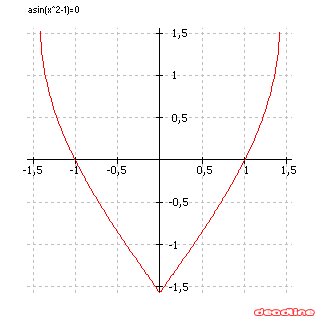# Solve trigonometric equations

Problem: solve a trigonometric equation

Equation to solve: asin(x^2-1) = 0

Interval for finding roots: [-pi/2, pi/2]

DeadLine can solve trigonometric equations instantly, providing the equation graph and the roots.

Number of roots found: 2

 x1 -1 x2 1